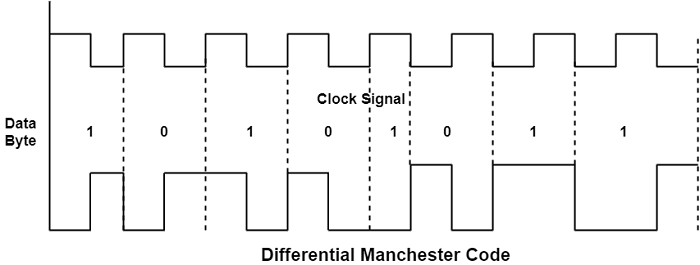# What is Polar Encoding?

Polar encoding is an encoding design that needs two voltage methods — one is positive, and the second is negative.

An average voltage method is decreased using both techniques, and the unipolar encoding scheme’s DC component is removed.

In Manchester and differential encoding, each bit includes both +ve and –ve voltage. Therefore, the DC component removed.

## Types of Polar Encoding

There are two types of Polar Encoding which are as follows −

### NRZ

NRZ represents Non-Return to Zero Code. In this coding, the signal level remains consistent during a bit duration.

There are three approaches used in NRZ are

In this coding system, the bit id represented by a voltage level. This voltage level remains constant during bit duration, as shown in the figure for data 110010111.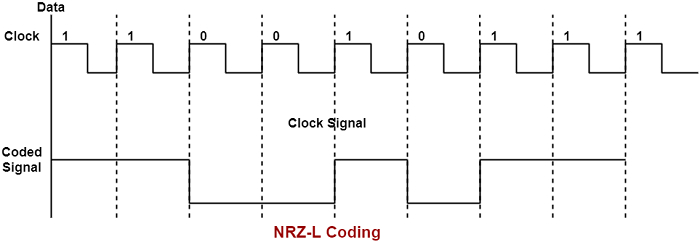In this encoding, '1' stands for mark and '0' for space. The signal level changes only for mark, i.e., for '1'. The figure shows an NRZ-M code for data 11001011.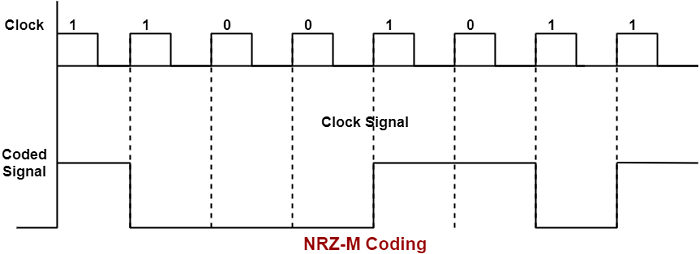This encoding is very much similar to the non-return to zero marks. The only change is that the signal changes only for space, i.e., for '0' and remain constant for mark, i.e., '1'. The figure shows the NRZ-S code for the data 11001011.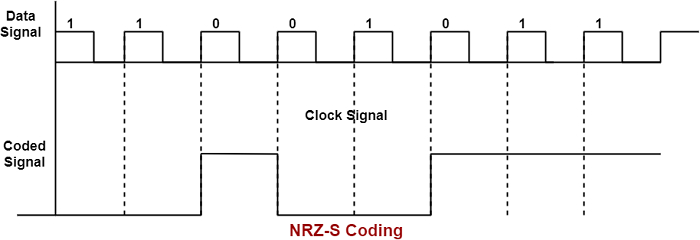### RZ

RZ stands for Return to zero Code. In digital transmission, the clock can be extracted if bits are continuously transmitted. If the data contains a continuous string of zeroes or ones, then in non-return to zero codes, the electrical signals will not have any level transition. A level change will not be there in codes.

The return to zero codes overcome the above limitations of NRZ codes. These codes are the combination of NRZ-L code and a clock signal.

There are four types of return to zero codes which are as follows

### Manchester Code

A negative to positive describes binary '1', and a positive to negative transitions shows binary '0'. So whatever be the bit sequence, each bit duration will have one transition, i.e., change-of-level. So at receiving end, the clock circuit faces no problems regarding level transitions.

This code is widely used in Local Area Network (LANs). It is also known as Biphase-L code, and an example for data bit 00110010 is shown in the figure −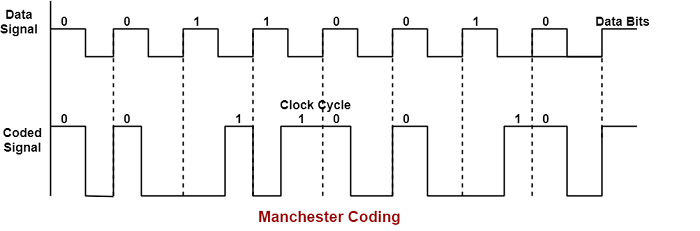### Biphase–M Code

In this coding system, there is continually a transition at the starting of the bit interval. The mark, i.e., binary '1', has an extra transition in the bit interval center.

The figure shows an example of data bit 11001011 using Biphase-M code −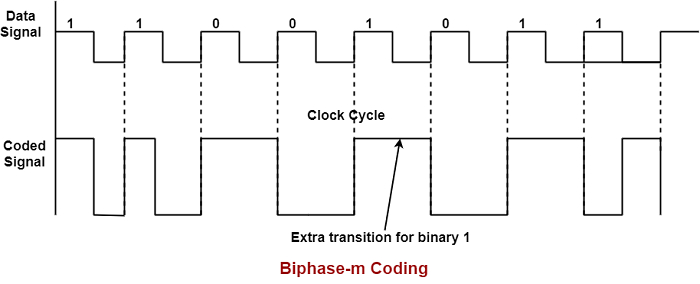### Biphase –S Code

This coding system is similar to the Biphase-M code system. There is continually a transformation at the starting of the bit interval. The only alteration is that space, i.e., '0', has an extra transition in the middle of the bit interval except for '1' as in Biphase-M code.

The figure shows an example of data bit 11001011 using BIphase-S Code −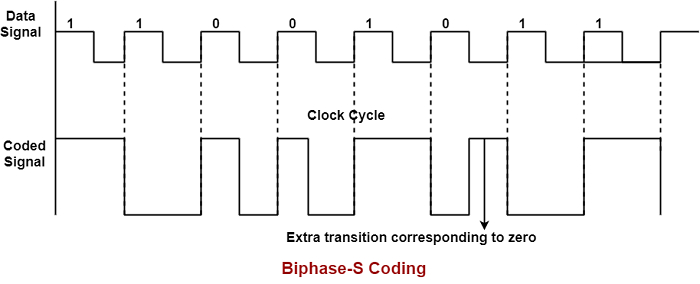### Differential Manchester Code

This coding system is continually a change in the middle of the bit interval. Space or binary '0' has an additional transition at the starting of the bit interval.

The figure shows an example of data bit 01001011 using differential-Manchester code.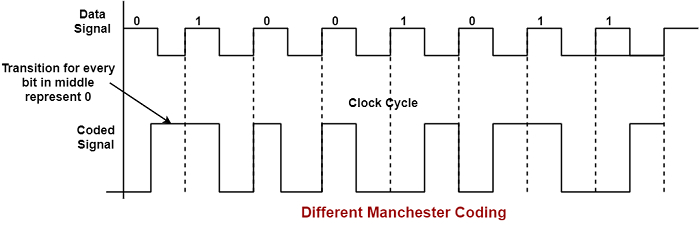Example 1 − Show the NRZ-L code for data byte 10110100

Solution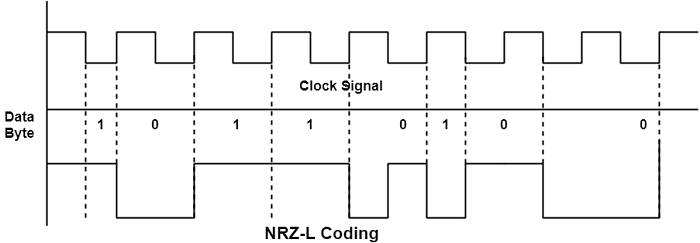Example 2 − Show the NRZ-S code for data byte 00010100

Solution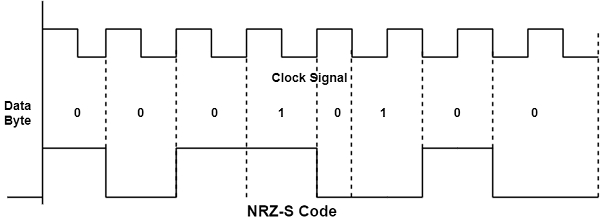Example 3 − Show the NRZ-M code for data byte 00111110

Solution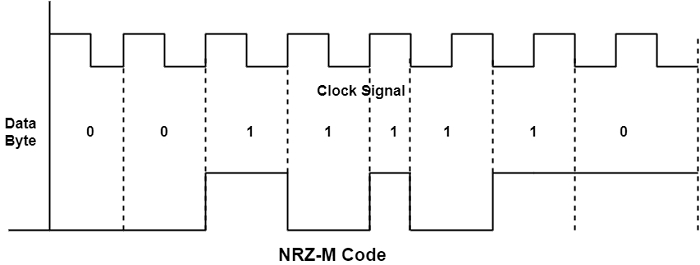Example 4 − Show the differential Manchester coding for data bytes 10101011

Solution